# Insertion Sort In Java Example | Java Insertion Sort Program

Here, we will see how can we sort our elements using a simple algorithm. Insertion sorting is less efficient on the large lists in comparison to others like Quicksort, MergeSort, etc. But it is efficient for simple sets of data. Example: quadratic sorting algorithms. We have already seen the QuickSort and Selection Sort in Java.

## Insertion Sort In Java

Insertion sort is the simple sorting algorithm that works in such a way that we are sorting play cards in our hands. We start with an empty left side, and the cards laid down on the table.

We then remove one card at a time from the table and insert it into the correct position in the left hand. To find the correct position for a new card, we compare it with the already sorted set of cards in hand, from right to left.

What Insertion sort does is that it removes an item from the array.

Then it compares it against the largest value in the array moves the element to its correct location.

## #Algorithm of Insertion Sort

See the following algorithm.

```INSERTION_SORT (A, N)(Array starts from O)
Repeat Step 2 to 4 for K = 1, 3, ..., N-1:
Set TEMP = A[K] and PTR = K - 1.
Repeat while TEMP < A[PTR] and PTR>=O:
(a) Set A[PTR+1] = A[PTR]
(b) Set PTR = PTR - 1.
[End of Loop.]
Set A[PTR+1] = TEMP.
[End of Loop 2.]
5. Return.```

Now let’s understand through an example:

Suppose we have given an array { 7, 4, 5, 2 }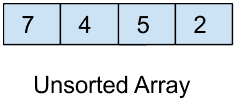### #Step: 1

We will start with the second element of the array that is 4 and checks whether it is smaller or greater than the first element that is 7. As it is smaller than 7 so put it in before 7.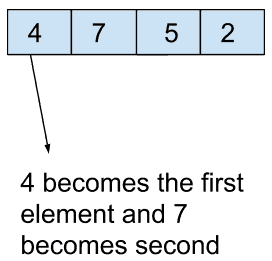### #Step: 2

Now move on the third element that is 5 and check whether it is greater or not than 7 as it is smaller so 5 becomes our second element and 7 becomes third and then check the second element with the first element that is 5 with 4 as 5 is greater than 4 so no change, and our array will be the following.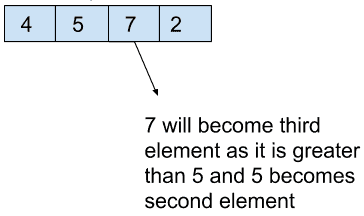### #Step: 3

Now move an item by item in the array and reach the last item as the last element is 2 so check it with the remaining elements from right to left as 2 is smaller than all the elements so change its position one by one with all the elements and in this way it gets reach to its correct place.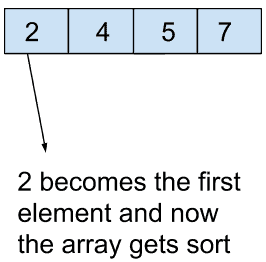In this way, we have got a sorted array that is { 2, 4, 5, 7}.

See the following code example.

```// InsertionSort.java

class InsertionSort {

void sort(int ar[]) {
int n = ar.length;
int k, i, j;
for (i = 1; i < n; i++) {
k = ar[i];
j = i - 1;
while (j >= 0 && ar[j] > k) {
ar[j + 1] = ar[j];
j = j - 1;
}
ar[j + 1] = k;
}
}

public static void main(String args[]) {
int ar[] = { 7, 4, 5, 2 };
int n = ar.length;
InsertionSort is = new InsertionSort();
is.sort(ar);
System.out.println("Array after Insertion Sort: ");
for (int i = 0; i < n; ++i)
System.out.print(ar[i] + " ");
System.out.println();
}
}```

See the following output.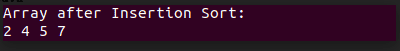## #Time Complexity

The time complexity of the Insertion sort is O(n*2).

And the auxiliary space used is O(1).

## #Applications

Insertion is mostly used in that place where the array was almost sorted, and the elements present in the array are small in number. Only in that place, Insertion Sort works efficiently; otherwise, other techniques are more efficient to work.

As the time complexity, in that case, is minimum and if the array is in reverse order, then insertion sort takes maximum time and hence time complexity increases.

Online: Insertion sort can sort a list as it receives it.

We can also use the binary search technique so that we have to make less comparison which we do in common insertion technique.

Standard Insertion Sorting takes O(i), but we can reduce it to O(log i) in the binary search technique.

## #What is Binary Insertion Sort

We can use the binary search to reduce the number of comparisons in the normal insertion sort.

Binary Insertion Sort uses the binary search to find a proper location to insert the selected element at each iteration.  In regular insertion, sorting takes O(i) (at ith iteration) in the worst case.

We can reduce it to an O(logi) by using a binary search.  The algorithm, as a whole, still has a running worst-case running time of O(n2) because of the series of swaps needed for each insertion.

Finally, Insertion Sort In Java Example is over.

This site uses Akismet to reduce spam. Learn how your comment data is processed.